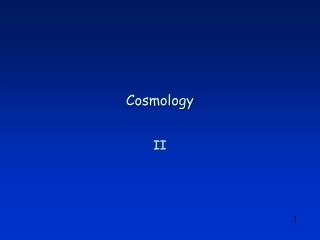Download PresentationCosmologyCosmology - PowerPoint PPT Presentation

Download PresentationCosmology
An Image/Link below is provided (as is) to download presentation

Download Policy: Content on the Website is provided to you AS IS for your information and personal use and may not be sold / licensed / shared on other websites without getting consent from its author. While downloading, if for some reason you are not able to download a presentation, the publisher may have deleted the file from their server.

- - - - - - - - - - - - - - - - - - - - - - - - - - - E N D - - - - - - - - - - - - - - - - - - - - - - - - - - -
Presentation Transcript

1. Topics • Models of the Universe: Recap • How Far is Far? • The Accelerating Universe • Summary

2. Models of the Universe – Recap Alexander Friedman 1888 - 1925 Density parameters are present epoch WM = rM / rc Matter WL = rL / rc Dark energy WM = WN + WD WN Normal matter WD Dark matter Friedman Equation Einstein’s equations for a flat geometry, no radiation

3. Models of the Universe – Recap – II Georges Lemâitre 1927 Assume WL= 0 a(0) = 0 Define a(t0) = 1 Solution Implies t0 ~ 10 Gyr for H0 = 70 km/s / Mpc

4. d0, t0 L = c (t0 – t1) d1, t1 How Far Is Far ? t0 – t1 is the look-back time d0 = d(t0) is the proper distance between the two galaxies now d1 = d(t1) is the proper distance between the two galaxies then

5. How Far is Far ? – II The Cartwheel Galaxy is said to be 500 million light years away. This, however, is not the distance to the Cartwheel now! It is merely the distance traveled by the light we are now receiving. So how far away is the Cartwheel now? t = t0 d0 a = 1

6. t = t1 t = t0 a < 1 d1 a = 1 How Far is Far ? – III We know t0 – t1 and t0 and we know that d(t) = a(t) d0. d0 Therefore, if we knew d0, we could compute the proper distance d(t) for any cosmic time t. By convention, the proper distances now define a reference frame called the comoving frame. This reference frame expands with the Universe, so comoving distances do not change. But the comoving distance coincides with the proper distance d(t) for our epoch t0.

7. t = t1 t = t0 a < 1 d1 a = 1 How Far is Far ? – IV d0 For light ds = 0, therefore For light ds = 0, therefore To compute d0 we note that for every increment of distance cdt traveled by light, the amount by which space expands is cdt / a(t). Therefore, using a(t) = (t/t0)2/3

8. t = t1 a < 1 d1 How Far is Far ? – V Light from the Cartwheel Galaxy has taken 500 million years to reach us, so, using t0 = 15 Gyr, t1 = 15 Gyr - 0.5 Gyr = 14.5 Gyr One finds d0 = 506 million ly But at time t1, the Cartwheel was at a distance of d1 = a(t1) d0 = (t1 / t0)1/3 x 506 million ly = 494 million ly t = t0 d0 a = 1

9. The Accelerating Universe • Measuring Using Standard Candles • Type Ia supernovae, which occur a few times per minute somewhere in the known Universe, can be used as standard candles to measure very great distances. • In 1998, two teams – one led by Saul Perlmutter, the other by Brian Schmidt – announced that these supernovae were fainter (and therefore further away) than expected for a decelerating Universe. • The Universe appears to be undergoing an acceleration by an anti-gravity effect such as that postulated by Einstein, but considered by him to be his biggest mistake.

10. Results • Matter Density • WM = 0.25 ± 0.08 • Dark Energy Density • WL = 0.75 ± 0.08 Scale factor for constant dark energy

11. Summary • Distances In an Expanding Universe • There are at least two: 1) the distance that light has traveled, which depends on two times t1 and t0 or 2) the proper distance, which depends on a single time. • Anti-Gravity • Distant Type Ia supernovae seem to be further away than expected. If this is confirmed, the implication is that the Universe is not slowing down as much as expected – and that some form of anti-gravity is countering the breaking effect of gravity.